NEET  >  NEET Previous Year Questions (2014-22): Oscillations

# NEET Previous Year Questions (2014-22): Oscillations - Notes | Study Physics Class 11 - NEET

 1 Crore+ students have signed up on EduRev. Have you?

Q.1. Two pendulums of length 121 cm and 100 cm start vibrating in phase. At some instant, the two are at their mean position in the same phase. The minimum number of vibrations of the shorter pendulum after which the two are again in phase at the mean position is:
(1) 9
(2) 10
(3) 8
(4) 11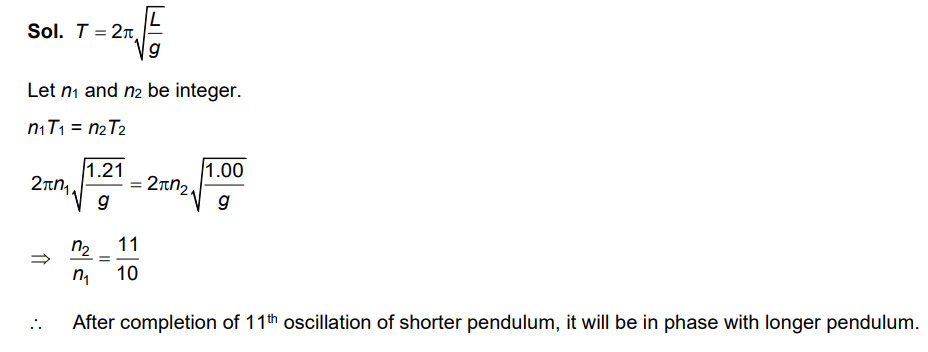Q 2. A body is executing simple harmonic motion with frequency 'n', the frequency of its potential energy is:    
A: 3n
B: 4n
C: n
D: 2n
Ans:
D
Solution:
Displacement equation of SHM of frequency 'n' x = Asin(ωt) = Asin(2πnt) Now,So frequency of potential energy = 2n

Q 3. A spring is stretched by 5 cm by a force 10 N. The time period of the oscillations when a mass of 2 kg is suspended by it is:     
A: 3.14 s
B: 0.628 s
C: 0.0628 s
D: 6.28 s
Ans:

Solution:

F = kx
10 = k(5 × 10–2)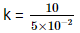= 2 x 102
= 200 N/m
Now,Q.4. Average velocity of a particle executing SHM in one complete vibration is:    
A: Aω/2
B: Aω
C: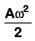D: Zero
Ans:
D
Solution:
In one complete vibration, displacement is zero. So, average velocity in one complete vibration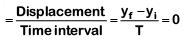Q 5. The displacement of a particle executing simple harmonic motion is given by y = A0+ Asinωt + Bcosωt
Then the amplitude of its oscillation is given by :    
A: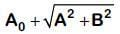B: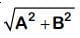C: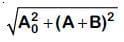D: A + B
Ans:
B
Solution: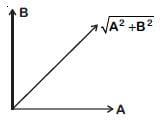y = A0+ Asinωt + Bsinωt
Equate SHM
y' = y – A0 = Asinωt + Bcosωt
Resultant amplitude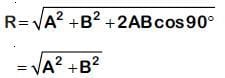Q 6. The radius of circle, the period of revolution, initial position and sense of revolution are indicated in the fig.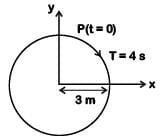y - projection of the radius vector of rotating particle P is:    
A: y(t) = –3 cos2πt, where y in m
B: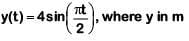C: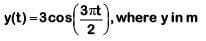D: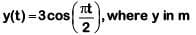Ans:
D
Solution:

At t = 0, y displacement is maximum, so equation will be cosine function.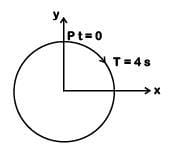T = 4s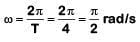y = a cosωt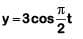Q 7. A pendulum is hung from the roof of a sufficiently high building and is moving freely to and fro like a simple harmonic oscillator. The acceleration of the bob of the pendulum is 20 m/s2 at a distance of 5 m from the mean position. The time period of oscillation is:-    
A: 2πs
B: πs
C: 2s
D: 1s
Ans:
B
Solution: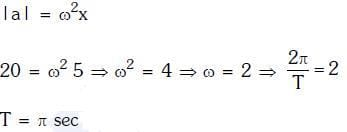Q 8. A particle executes linear simple harmonic motion with an amplitude of 3 cm. When the particle is at 2 cm from the mean position, the magnitude of its velocity is equal to that of its acceleration. Then its time period in seconds is:-    
A: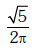B: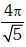C: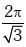D: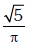Ans:
B
Solution: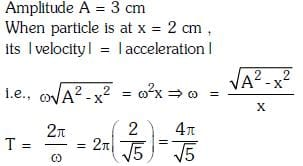Q 9. A particle is executing SHM along a straight line. Its velocities at distances x1 and x2 from the mean position are V1 and V2, respectively. Its time period is:    
A: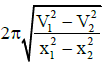B: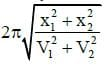C: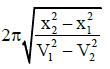D: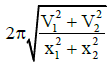Ans: C
Solution: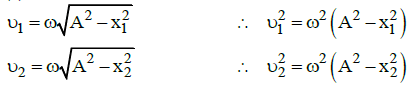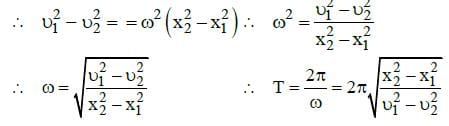Q 10. When two displacements represented by y1 = a sin(ωt) and y2 = b cos(ωt) are superimposed the motion is:    
A: simple harmonic with amplitude (a+b)/2
B: not a simple harmonic
C: simple harmonic with amplitude a/b
D: simple harmonic with amplitude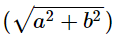Ans:
D
Solution: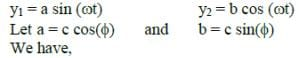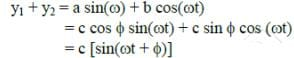where  c2 = a2 + b2  [since  a+ b2 = c2 cos (φ) + csin (φ) = c2 ]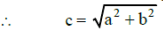The superimposed motion is simple harmonic with amplitude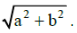Q 11. The oscillation of a body on a smooth horizontal surface is represented by the equation,
X = A cos (ωt)
Where X = displacement at time t
ω = frequency of oscillation
Which one of the following graphs shows correctly the variation ‘a’ with ‘t’?    
A: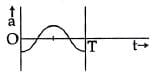B: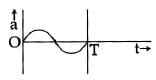C: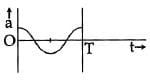D: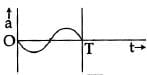Here, a = acceleration at time ‘t’ and T = time period
Ans:
A
Solution: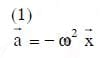The document NEET Previous Year Questions (2014-22): Oscillations - Notes | Study Physics Class 11 - NEET is a part of the NEET Course Physics Class 11.
All you need of NEET at this link: NEET

## Physics Class 11

127 videos|464 docs|210 tests
 Use Code STAYHOME200 and get INR 200 additional OFF

## Physics Class 11

127 videos|464 docs|210 tests

### How to Prepare for NEET

Read our guide to prepare for NEET which is created by Toppers & the best Teachers

Track your progress, build streaks, highlight & save important lessons and more!

,

,

,

,

,

,

,

,

,

,

,

,

,

,

,

,

,

,

,

,

,

;